# Simultaneous inference for Best Linear Predictor of the Conditional Average Treatment Effect and other structural functions

4 July 2018

### Type

Working Paper (CWP40/18)

### DOI

This paper provides estimation and inference methods for a structural function, such as Conditional Average Treatment Effect (CATE), based on modern machine learning (ML) tools. We assume that such function can be represented as a conditional expectation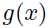=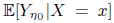of a signal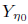, where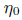is the unknown nuisance function. In addition to CATE, examples of such functions include regression function with Partially Missing Outcome and Conditional Average Partial Derivative. We approximateby a linear form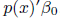, where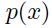is a vector of the approximating functions and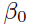is the Best Linear Predictor. Plugging in the first-stage estimateinto the signal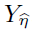, we estimatevia ordinary least squares ofon. We deliver a high-quality estimate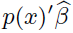of the pseudo-target function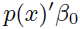, that features (a) a pointwise Gaussian approximation of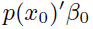at a point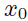, (b) a simultaneous Gaussian approximation ofuniformly over x, and (c) optimal rate of convergence oftouniformly over x. In the case the misspecication error of the linear form decays sufficiently fast, these approximations automatically hold for the target functioninstead of a pseudo-target. The first stage nuisance parameteris allowed to be high-dimensional and is estimated by modern ML tools, such as neural networks,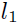-shrinkage estimators, and random forest. Using our method, we estimate the average price elasticity conditional on income using Yatchew and No (2001) data and provide uniform confidence bands for the target regression function.